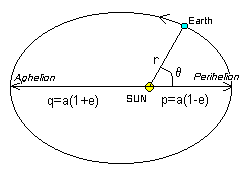### The Earth's Orbit Around the Sun

The Earth moves around the Sun in an orbit that is almost but not quite circular. As Kepler proved in the seventeeth century, the orbit is actually an ellipse. A parameter called the eccentricity (e) defines the degree of departure from a circle. A value of e=0 would indicate a circle whereas a value of e=0.9 would indicate a very elongated ellipse. The eccentricity of the Earth's orbit is currently e=0.0167. The diagram belows shows the details of the Earth's elliptical orbit very much exaggerated.In this view we are looking from above the north pole of the Sun (and the Earth). The Earth moves in an anticlockwise motion in this view. The distance from the Earth to the Sun is denoted by the symbol r at any time. This can vary from the perihelion distance p (when the Earth is at its closest point to the Sun) to the aphelion distance q (when the Earth is at its furthest point from the Sun).

In this current epoch, the Earth passes through perihelion early in January, and through aphelion early in July. The symbol a (the semimajor axis of the ellipse) denotes the mean distance of the Earth from the Sun and is a fundamental unit of astronomical distance measurement called the Astronomical Unit. It has a value of 1 AU = 149,597,870 km. Using the quoted value for the eccentricity we can compute values for the perihelion and aphelion distances of p=0.983 AU and q=1.017 AU. Thus the Earth's distance from the Sun changes by about 3 % over the year.

This distance variation has consequences in both optical and radio solar observations. The apparent diameter of the Sun changes by about 3%, and the measured solar radio flux changes as the inverse square of the distance. Background solar fluxes are normally reported as fluxes corrected to a distance of 1 AU and may need to be uncorrected if used for equipment calibration purposes.